# How to Use the Excel SUMPRODUCT FunctionClaudia Buckley#### Join the Excel conversation on Slack

Ask a question or join the conversation for all things Excel on our Slack channel.

Do you know the SUMPRODUCT function in Excel

If not, what you don’t know can cost you in terms of time and effort because Excel SUMPRODUCT is a powerful, but somewhat less-used function. It has been around since the earliest versions of Excel, and while much of its basic usage has been replaced by newer dynamic array functionality, we're not ready to get rid of it just yet.

## How to use SUMPRODUCT

By default, the SUMPRODUCT function multiplies values within ranges or arrays, then finds the sum of those products. However, the formula can be modified to perform addition, subtraction, or division operations instead.

SUMPRODUCT is unlike most other functions because it behaves like an array function without having to press Control + Shift + Enter (CSE). Array functions perform multiple calculations on a value or values within a row or column, all in one entry.

Use this free Excel sumproduct file to practice along with the tutorial.

### Syntax

To use the default operation (multiplication), the syntax is:

````=SUMPRODUCT(array1, [array2], [array3], ...)`
```

### Arguments

• Array1 is the first range or array to be multiplied.
• Array2 is the second range or array to be multiplied. Array2 and all subsequent arrays are optional.

### Return value

SUMPRODUCT returns a single value which is the sum of the product of the arrays used in the formula.

### Basic use

The basic use of SUMPRODUCT is illustrated in the example below.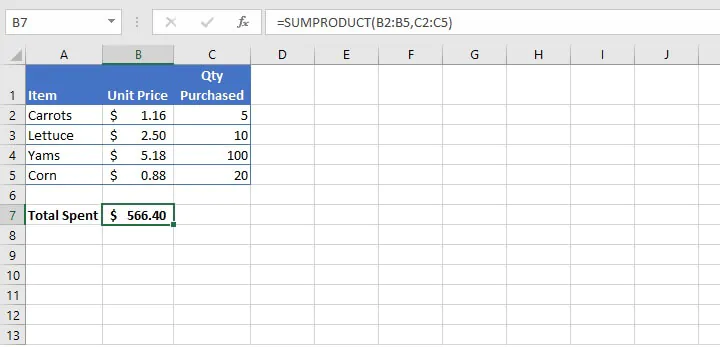To calculate the amount spent on the grocery items above, the usual way to perform this manually would be to expand each row by multiplying B2 by C2, B3 by C3, and so on, placing the results in column D. Once the amount paid for each product has been calculated, we would then find their total.

Excel simplifies this entire process with SUMPRODUCT. The same multiplication operations are performed in the background: (\$1.16 * 5), (\$2.50 * 10), (\$5.18*100), (\$0.88 * 20). But the results are stored in memory, and then added together to get the grand total of \$566.40.

### Remarks

• If only one array is used, SUMPRODUCT will simply add all the values in the array.
• Arrays must be of the same size for the SUMPRODUCT function to work.
• If any array argument contains non-numeric values, they will be treated as zeros.
• It isn’t a good idea to use SUMPRODUCT with full column references (for example, A:A or B:B). Since Excel columns have over one million rows, you’d be asking Excel to scan through all those rows, which unnecessarily slows down the performance of your worksheet.

## Perform other arithmetic operations

To use SUMPRODUCT to perform division, addition, or subtraction on arrays separate each argument with the appropriate arithmetic symbol (/, +, -) for the operation required. After all the operations have been performed, the results are summed as usual.

For example:

``=SUMPRODUCT(A1:A3/B1:B3)``

will divide the value in A1 by the value in B1, the value in A2 by the value in B2, and the value in A3 by the value in B3, and add the results.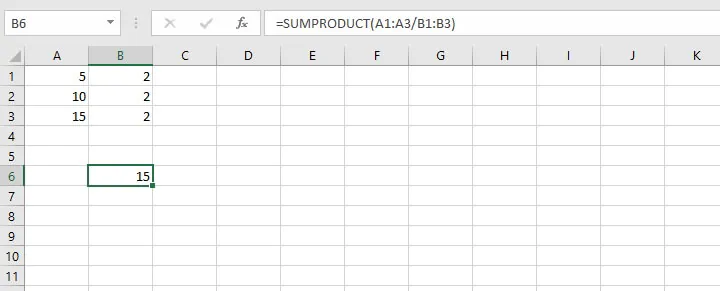Performing a subtraction operation would apply the same principle.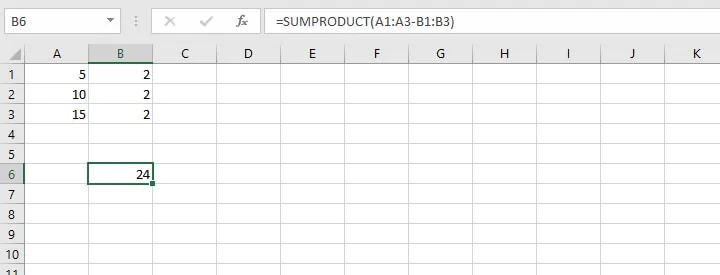Of course, if other arrays are introduced, it’s a good idea to use parentheses so that the operations are performed in the desired order.

For example:

``=SUMPRODUCT(A1:A3-B1:B3*B1:B3)``

produces a different result from SUMPRODUCT((A1:A3-B1:B3)*B1:B3)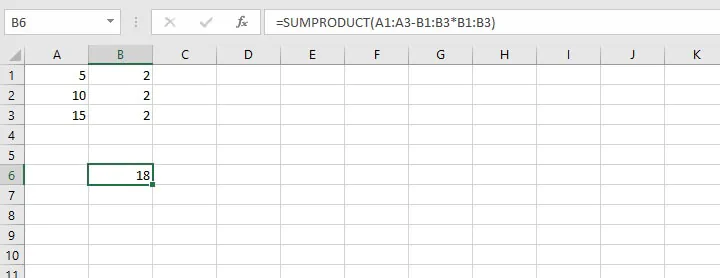In the above formula, Excel performed the multiplication operation first, following the PEMDAS (Parentheses – Exponents – Multiplication – Division – Addition – Subtraction) order of operations rule.

The multiplication of the arrays B1:B3 and B1:B3 was carried out first, then the resulting values were subtracted from A1:A3, to return a value of 18.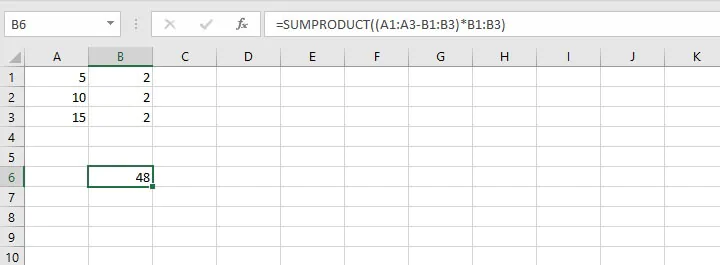When the formula is written as above, Excel performs the subtraction equation within parentheses first, then multiplies the resulting values by the B1:B3 array, giving a result of 48.

## Calculate weighted average

A fairly common application of SUMPRODUCT is to calculate the weighted average using the following format: SUMPRODUCT(values, weights) / SUM(weights)

Values may be assigned certain weights based on their priority, or the “weight” given to values which total 100%.

In the following example, various weights have been assigned to each test to determine the final score for that subject.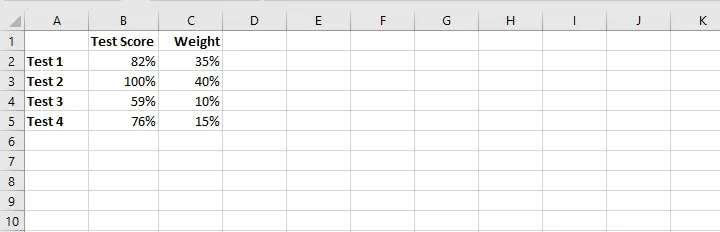Without SUMPRODUCT, this problem would likely be solved by multiplying each score by its respective weight and then adding those results to arrive at the overall grade.

Using the SUMPRODUCT format stated above reduces those calculations to a single calculation in Excel.

``=SUMPRODUCT(B2:B5,C2:C5)/SUM(C2:C5)``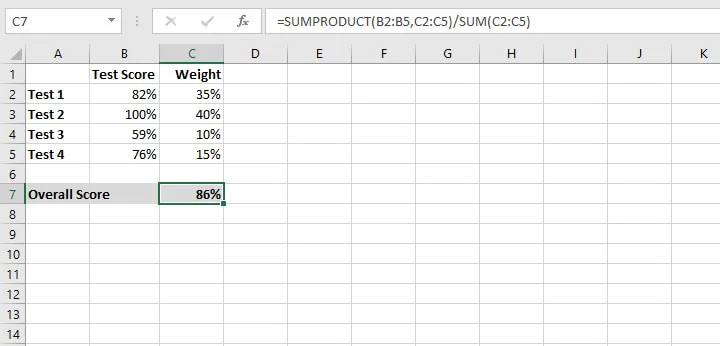SUMPRODUCT can be used in creative ways to do what other functions can do. This is particularly useful when some of these functions aren’t available in the version of Excel you’re using. A couple of examples are shown below.

## Count cells that match multiple criteria without COUNTIFS

The COUNTIFS function was released in Excel 2007, so we have grown accustomed to having it match cells that meet multiple criteria. But if you have ever found yourself using an older version of Excel, you might have resorted to nesting several COUNTIF functions within each other. This can get a bit tricky if there are many conditions in your formula.

SUMPRODUCT can handle that using the following syntax:

``=SUMPRODUCT((range1="criteria1")*(range2="criteria2"))``

For example, in the following data set, there is a Sales Rep named Josh on Team A, and there is also a Josh on Team B.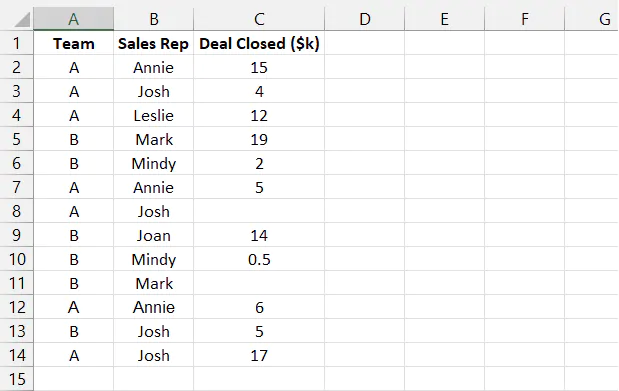To count the number of deals closed by Josh on Team A, we need to specify three conditions:

1. Criteria "A" in column A

2. Criteria "Josh" in column B

3. Criteria nonblank in column C.

``=SUMPRODUCT((A2:A14="A")*(B2:B14="Josh")*(C2:C14<>""))``

##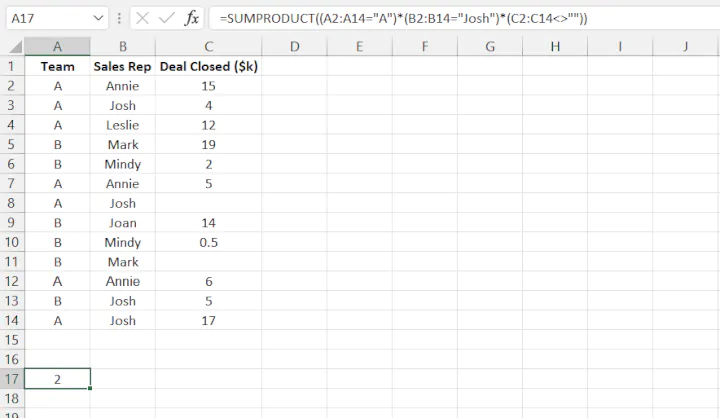Note that the <> symbol representing "not equal to" is used to replace the equal sign (=) in the syntax.

## Count distinct values in Excel without UNIQUE

The UNIQUE function in Excel is designed to either count the number of distinct values in an array, or it can count the number of values appearing exactly once. The idea of ‘distinct’ values essentially answers the question, “How many different values are referred to in this list, removing duplicates?”

However, since the UNIQUE function is only available in Excel 365 and Excel online, here is a workaround, using SUMPRODUCT.

The SUMPRODUCT can be used to count distinct values with the format:

``=SUMPRODUCT(1/COUNTIF(range, range))``

Using this format to count the number of distinct city names from the list of 17 cities in the range below, we would enter:

``=SUMPRODUCT(1/COUNTIF(B2:B18, B2:B18))``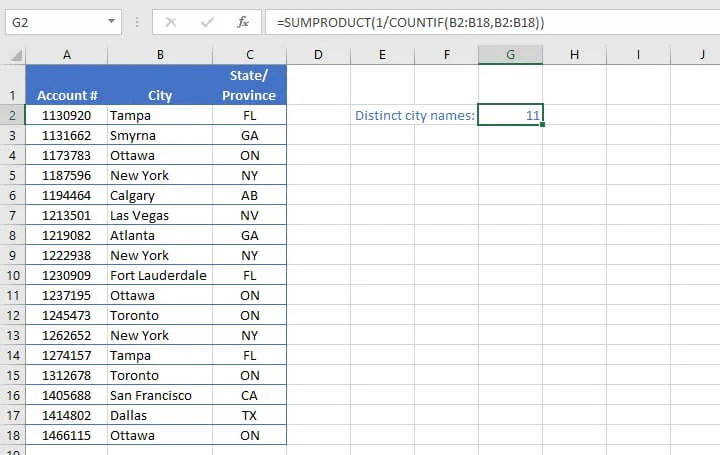The result returned is that there are 11 distinct city names. A manual check reveals that this is true, but let’s break the formula down to see how Excel got that result. We’ll start with the inner section of the formula, COUNTIF.

``COUNTIF(B2:B18, B2:B18)``

As you may already know, we use the COUNTIF function to find out how many times each individual value appears in the specified range. By selecting the range B2 to B18 as both the reference range and the criteria range, we are asking Excel to count how many times each value appears in the array.

The result in our example would be: {2,1,3,3,1,1,1,3,1,3,2,3,2,2,1,1,3}. Excel stores this array in its memory, and uses that to process the section of the formula which says:

``1/COUNTIF(B2:B18, B2:B18)``

This simply divides the number 1 by each value from the COUNTIF result in turn. If you take a moment to think about this, what will happen is that values within the array that are equal to 1 will return a value of 1 when divided by 1.

However, values that are equal to 2 will return a value of 0.5 when divided by 1. Therefore, both values which appear twice in the data set would total 1. This accomplishes our task of counting each distinct value only once.

The SUMPRODUCT portion of the formula:

``SUMPRODUCT(1/COUNTIF(B2:B18, B2:B18))``

then looks at the results as an array to be added, thereby returning a result of 11 — the total number of all the distinct values within the original data set.

## Using SUMPRODUCT to unlock other functions

The array-type functionality of SUMPRODUCT can be used to help ordinary functions to do extraordinary things.

For example, the LEN function, used to count the number of characters in a text string, can only be used for a single cell reference. But what if the values to be counted were spread across several cells, such as in the case below?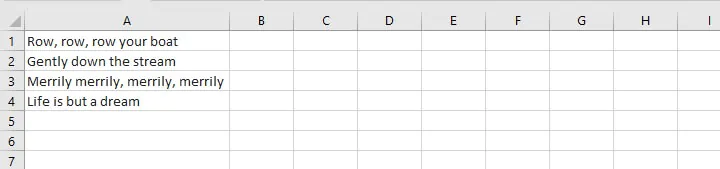The ordinary way to count the number of characters in this range might be to use the LEN function in cells B1, B2, B3, and B4 and then total those numbers.

``LEN(A1)``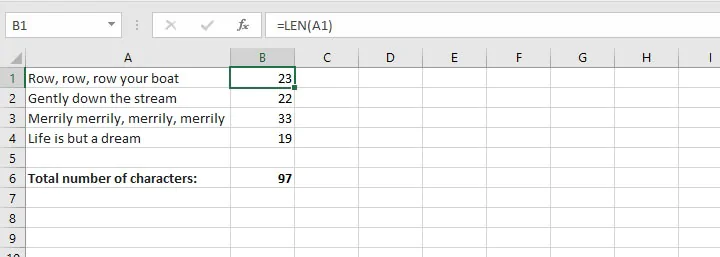You might even think of accomplishing the same thing by using a single entry, like this:

``=SUM(LEN(A1),LEN(A2),LEN(A3),LEN(A4))``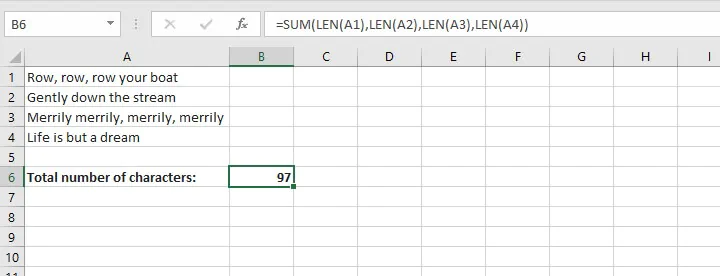But did you think of using SUMPRODUCT like this:

````=SUMPRODUCT(LEN(A1:A4))`
```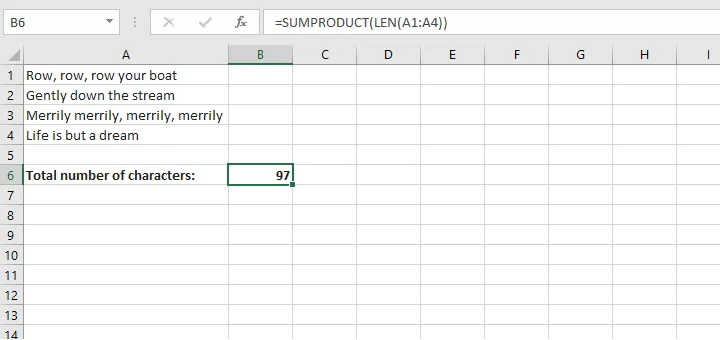Combining SUMPRODUCT with LEN allows you to enter a range of cells, which is not a feature of the standard LEN function.

The result of each cell is added since this functionality is built into SUMPRODUCT. This brings the total to 97 without having to use Control + Shift + Enter or Control + Enter.

These are just a few examples of how the Excel SUMPRODUCT function can help you get even more out of Excel.

Check out our comprehensive Excel - Basic and Advanced course.

Ready to become a certified Excel ninja?